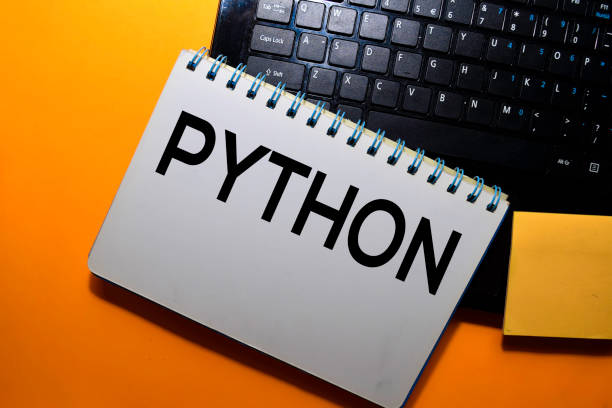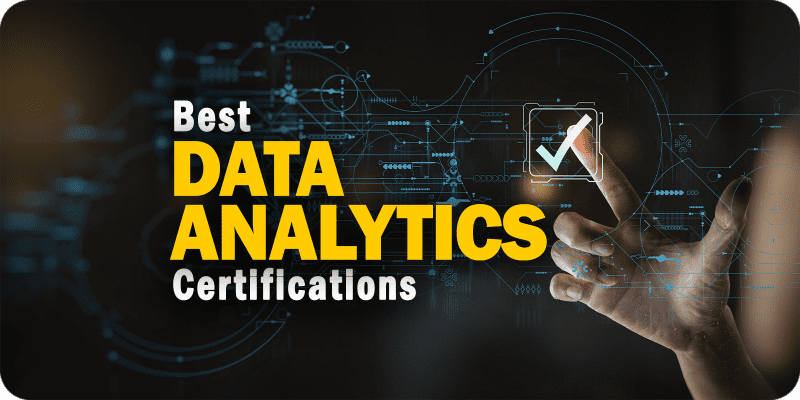# Data Science Training in Vizag

Become a Data Scientist and learn Statistical Analysis, Machine Learning, Predictive Analytics, and many more.## Data Science Certification Programme Overview

This Data Science Course using Python and R endorses the CRISP-DM Project Management methodology and contains a preliminary introduction of the same.

### Python Certification Training Course in Visakhapatnam

Data Science is a 90% statistical analysis and it is only fair that the premier modules should bear an introduction to Statistical Data Business Intelligence and Data Visualization techniques. Students will grapple with Plots, Inferential Statistics, and various Probability Distributions in the module.

A brief exposition on Exploratory Data Analysis/ Descriptive Analytics is huddled in between. The core modules commence with a focus on Hypothesis Testing and the “4” must know hypothesis tests.

Data Mining with Supervised Learning and the use of Linear Regression and OLS to enable the same find mention in succeeding modules.

The prominent use of Multiple Linear Regression to build Prediction Models is elaborated.

The theory behind Lasso and Ridge Regressions, Logistic Regression, Multinomial Regression, and Advanced Regression For Count Data is discussed in the subsequent modules.## Data Science Training in Vizag

What is Data Science?

Data science is an amalgam of methods derived from statistics, Data Analysis, and Machine Learning that are trained to extract and analyze huge volumes of structured and unstructured data.

Who is a Data Scientist?

A Data Scientist is a researcher who has to prepare huge volumes of big data for analysis, build complex quantitative algorithms to organize and synthesize the information, and present the findings with compelling visualizations to senior management.

A Data Scientist must be a person who loves playing with numbers and figures. A strong analytical mindset coupled with strong industrial knowledge is the skill set most desired in a data scientist.

He must possess above the average communication skills and must be adept in communicating the technical concepts to non – technical people.

Data Scientists need a strong foundation in Statistics, Mathematics, Linear Algebra, Computer Programming, Data Warehousing, Mining, and modeling to build winning algorithms.

They must be proficient in tools such as Python, R, R Studio, Hadoop, MapReduce, Apache Spark, Apache Pig, Java, NoSQL database, Cloud Computing, Tableau, and SAS.## Data Science Training Learning Outcomes

The Data Science using Python and R commences with an introduction to statistics, probability, python and R programming, and Exploratory Data Analysis.

Participants will engage with the concepts of Data Mining Supervised Learning with Linear regression and Predictive Modelling with Multiple Linear Regression techniques.

Data Mining Unsupervised using Clustering, Dimension Reduction, and Association Rules is also dealt with in detail.

A module is dedicated to scripting Machine Learning algorithms and enabling Deep Learning and Neural Networks with Black Box techniques and SVM.

Learn to perform proactive forecasting and Time Series Analysis with algorithms scripted in Python and R. in the best data science training institute in India.

• Work with various data generation sources
• Perform Text Mining to generate Customer Sentiment Analysis
• Analyse structured and unstructured data using different tools and techniques
• Develop an understanding of Descriptive and Predictive Analytics
Apply Data-driven, Machine Learning approaches for business decisions
Build models for day-to-day applicability
Perform Forecasting to take proactive business decisions
Use Data Concepts to represent data for easy understanding

## Data Science Certification Modules

This data science program follows the CRISP-DM Methodology. The premier modules are devoted to a foundational perspective of Statistics, Mathematics, Business Intelligence, and Exploratory Data Analysis.

The successive modules deal with Probability Distribution, Hypothesis Testing, Data Mining Supervised, Predictive Modelling – Multiple Linear Regression, Lasso And Ridge Regression, Logistic Regression, Multinomial Regression, and Ordinal Regression.

Later modules deal with Data Mining Unsupervised Learning, Recommendation Engines, Network Analytics, Machine Learning, Decision Tree and Random Forest, Text Mining, and Natural Language Processing.

The final modules deal with Machine Learning – classifier techniques, Perceptron, Multilayer Perceptron, Neural Networks, Deep Learning Black-Box Techniques, SVM, Forecasting, and Time Series algorithms. This is the most enriching training program in terms of the array of topics covered.

## Python Introduction

• Introduction to Python Programming
• Installation of Python & Associated Packages
• Graphical User Interface
• Installation of Anaconda Python
• Setting Up Python Environment
• Data Types
• Operators in Python
• Arithmetic operators
• Relational operators
• Logical operators
• Assignment operators
• Bitwise operators
• Membership operators
• Identity operators
• Check out the Top Python Programming Interview Questions and Answers here.
• Data structures
• Vectors
• Matrix
• Arrays
• Lists
• Tuple
• Sets
• String Representation
• Arithmetic Operators
• Boolean Values
• Dictionary
• Conditional Statements
• if statement
• if – else statement
• if – elif statement
• Nest if-else
• Multiple if
• Switch
• Loops
• While loop
• For loop
• Range()
• Iterator and generator Introduction
• For – else
• Break
• Functions
• Purpose of a function
• Defining a function
• Calling a function
• Function parameter passing
• Formal arguments
• Actual arguments
• Positional arguments
• Keyword arguments
• Variable arguments
• Variable keyword arguments
• Use-Case *args, **kwargs
• Function call stack
• Locals()
• Globals()
• Stackframe
• Modules
• Python Code Files
• Importing functions from another file
• __name__: Preventing unwanted code execution
• Importing from a folder
• Folders Vs Packages
• __init__.py
• Namespace
• __all__
• Import *
• Recursive imports
• File Handling
• Exception Handling
• Regular expressions
• Oops concepts
• Classes and Objects
• Inheritance and Polymorphism

### SQL

• What is a Database
• Types of Databases
• DBMS vs RDBMS
• DBMS Architecture
• Normalisation & Denormalization
• Install PostgreSQL
• Install MySQL
• Data Models
• DBMS Language
• ACID Properties in DBMS
• What is SQL
• SQL Data Types
• SQL commands
• SQL Operators
• SQL Keys
• SQL Joins
• GROUP BY, HAVING, ORDER BY
• Subqueries with select, insert, update, delete
• atements?
• Views in SQL
• SQL Set Operations and Types
• SQL functions
• SQL Triggers
• Introduction to NoSQL Concepts
• SQL vs NoSQL
• Database connection SQL to Python
• Check out the SQL for Data Science One Step Solution for Beginners here.